# JNTU MEFA Notes for DEMAND ANALYSIS

Posted by Vishnureddy at 11:32 PMMANAGERIAL ECONOMICS AND FINANCIAL ANALYSIS
By: - B. Mallesham,
Assistant prof,
MBA dept, JITS.
DEMAND ANALYSIS – II

ELASTICITY OF DEMAND

Elasticity of Demand: - it is necessary to find out the extent of increase or decrease in each of the variables for taking certain managerial decisions. This passes the way for the concept of elasticity of demand.

Definition: - the rate of responsiveness in the demand of a commodity for a given change in price or any other determinants of demand.

MEASUREMENT OF ELASTICITY: -

The elasticity is measured in the following ways.

1. Perfectly elastic demand.

2. Perfectly inelastic demand.
3. Relatively elastic demand.
4. Relatively inelastic demand.
5. Unity elasticity.
1. Perfectly elastic demand:-
When any quantity can be sold at a given price, and when there is no need to reduce the price, the demand is said to be perfect elastic. Even a small increase in price will lead to complete fall in demand.
The quantity demanded increases from OQ to OQ1 from OQ1 to OQ2 even through there is no change in price. Price is fixed at OP.Price

0 q q1 q2 q3 quantity demanded.

2. Perfectly inelastic demand:-
When a significant degree of change in price leads to little (or) no change in the quantity demanded. Then the elasticity is said to be perfectly inelastic.
In other words, the demand is said to be perfectly inelastic when there is no change in the quantity demanded even though there is big change (increase or decrease) in price.

Price
P2P1

P

0 q quantity demanded.

From the above figure, there is no change in the quantity demanded though there is change in price, say increase or decrease. In other words, despite the increase in price from OP to OP1. The quantity demanded has not fallen down. Similarly, though there is a fall in price from OP3 TO OP2, the quantity demanded remains unchanged.

3. Relatively elastic demand:-
The demand is said to be relatively elastic when the change in demand is more than the change in the price.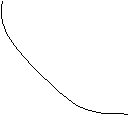D
Price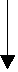P1P2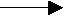0 Q1 Q2 quantity demanded

From the above figure, the quantity demanded increases from OQ1 to OQ2 because in price from OP1 to OP2. The extent of increase in the quantity demanded is greater than the extent of fall in the price.

4. Relatively inelastic demand:-
The demand is said to be relatively inelastic when the change in demand is less than the change in the price.D
Price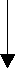P1P20 Q1 Q2 quantity demanded

From the above figure, the quantity demanded increases from OQ1 to OQ2, because of the decrease in price from OP1 to OP2. The extent of increase in the quantity demanded is lesser than the extent of fall in price.

5. Unity elasticity:-
The elasticity in demand is said to be unity when the change in demand is equal to the change in price.D
Price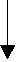P1P20 Q1 Q2 quantity demanded

From the above figure the quantity demanded increases from OQ1 to OQ2 because of the decrease in price from OP1 to OP2. The extent of increase in the quantity demanded is equal to the extent of fall in price.

TYPES OF ELASTICITY
There are 4 types of elasticity of demand
1. Price elasticity of demand.
2. Income elasticity of demand.
3. Cross elasticity of demand.

1. Price elasticity of demand:-
Elasticity of demand in general refers to the price elasticity of demand. In other words, it refers to the quantity demanded of a commodity in response to a given change in price. Price elasticity is always negative which indicates that the customer tends to buy more with every fall in the price. The relationship between the price & the demand in inverse.
It is measured as follows…..
Price elasticity of demand = Proportional change in the quantity demanded for product X
Proportional change in the price of X.
Edp= (Q2-Q1)/Q1/ (P2-P1)/P1.
Q1= quantity demanded before price change.
Q2= quantity demanded after price change.
P1= price before change.
P2= price after change.
The price is said to be elastic, when the proportionate change in quantity demanded is more than the proportionate change in price.
Ex: 5% fall in the price results in an increase of 20% in the quantity demanded, the price is said to be elastic, which implies that the elasticity is more than one (e>1).
On the other hand, if the price is said to be inelastic then it means that the quantity demanded is less than the proportional change in the price. A 10% increase in price resulting in a 2% drop in the quantity demanded of the profit implies a product where the demand is said to be price inelastic, which means the elasticity is less than one(e<1 span="">

Example 1: -
Elastic demand (e>1).
Determine the price elasticity
• The quantity demanded for product is 1000 units at a price of 100/-.
• The price declines to 90/- & the quantity demanded increases to 1500 units
Sol: -  Edp= (Q2-Q1)/Q1/(P2-P1)/P1
Q1=1000 units
Q2=1500 units
P1= 100/-
P2= 90/-
Edp= (1500-1000)/1000/(90-100)/100 = -5
Since Edp in -5,it means for a 10% change in price, there is a change in demand by 50% where the numerical value of elasticity is more than one the demand is elastic in other words, the % of increase in quantity demanded is more than the % change in decrease in price.

Example 2: -
Inelastic price demand (e<1 span="">
Determine the price elasticity
• The quantity demanded for product is 1000 units at a price of 100/-.
• The price declines to 70/- & the quantity demanded increases to 1100 units.
Sol: - Edp= (Q2-Q1)/Q1/(P2-P1)/P1
Q1=1000 units
Q2=1100 units
P1= 100/-
P2= 70/-
Edp= (1100-1000)/1000/(70-100)/100 = -0.33
Since Edp in -0.33, it means for a 10% fall in price, there is an increase in demand by 3.3%. Where the numerical value of elasticity is less than one, the price demand is inelastic. In other words, the % of increase in quantity demanded is less than the % of decrease in price.

Example 3:-
Unity price elasticity (e=1)
Determine the price elasticity
• The quantity demanded for product is 1000 units at a price of 100/-.
• The price declines to 50/- & the quantity demanded increases to 1500 units.
Sol: - Edp= (Q2-Q1)/Q1/(P2-P1)/P1
Q1=1000 units
Q2=1500 units
P1= 100/-
P2= 50/-
Edp= (1500-1000)/1000/(50-100)/100 = 1.0
Since Edp in 1, it means for a 50% fall in price, there is an increase in demand by 50%. Where the numerical value of elasticity is equal to one, the price demand is unity elastic. In other words, the % of increase in quantity demanded is less than the % of decrease in price.

Significance of price elasticity of demand -
It is necessary that the trader should be aware of the impact of changes in the quantity demanded for a given change in price. He can take a decision as to how much he can supply if he is aware of the likely change in quantity demanded as a result of change in price.

2. Income elasticity of demand:-
Income elasticity of demand refers to the quantity demanded of a commodity in response to a given change in income of the consumer.
Income elasticity is normally positive, which indicates that the consumer tends to buy more and more with every increase in income.
Income elasticity of demand= Proportional change in quantity demanded for product X
Proportional change in income.
Edi= (Q2-Q1)/Q1/ (I2-I1)/I1
Q1= quantity demanded before change,
Q2= quantity demanded after change.
I1= income before change.
I2= income after change.
Positive income elasticity indicates that the demand for the product rises more quickly them the rise in disposable income. In other words, the demand is more responsive to a change in income.

Example 4: -
Elastic income demand (e>1)
Determine the income elasticity
• The quantity demanded for product is 1000 units at a price of 100/-.
• The income declines to 80/- & the quantity demanded decreases to 700 units.
Sol: - Edp= (Q2-Q1)/Q1/ (I2-I1)/I1
Q1=1000 units
Q2=700 units
I1= 100/-
I2= 80/-
Edp= (700-1000)/1000/(80-100)/100 = 1.5
Since Edi in 1.5, it means for a 10% fall in income, there is a decrease in demand by 15%. Where the numerical value of elasticity is more than one, the price demand is relatively elastic. In other words, the % of decrease in quantity demanded is more than the % of fall in income. In times of depression, the incomes fall & consequently the demand for the goods & services also decrease.
Similarly the inelastic income demand and unity income demand can be determined.

Significance of income elasticity of demand:-
In determining the effects of changes in business activity it is necessary for the trader to be aware of the income elasticity of demand for given commodities. With the help pf income elasticity of demand, he can estimate the likely changes in the demand for his product as a result of changes in the national income. Income elasticity will help us in knowing whether a commodity is a superior good, normal good or an inferior good.
If the income elasticity is positive and greater than one, it is a superior good. The superior goods such as automobiles and refrigerators can be located where high income group customers find it convenient to shop. If the income elasticity is positive and less than or equal to one, it is a normal good. If the income elasticity is negative, it is an inferior good. Knowledge of the nature of goods help in allocating advertising budget.

3. Cross elasticity of demand:-
Cross elasticity of demand refers to the quantity demanded of a commodity in response to a change in the price of a related good, which may be substitute or compliment.

Cross elasticity of demand= proportional change in quantity demand for product X
Proportional changes in price of product X.
Edc= (Q2-Q1)/Q1/ (P2y-P1y)/P1y.
Q1= quantity demanded before change.
Q2= quantity demanded after change.
P1y= price before change
P2y= price after change.

Example 5: -
Inelastic cross demand (e<1 span="">
Determination of cross elasticity
• The quantity demanded for a product is 1000 units at a price of 20/-.
• The income increases to 30/- & the quantity demanded increases to 1200 units.
Sol: - Edp= (Q2-Q1)/Q1/(P2y-P1y)/P1y
Q1=1000 units
Q2=1200 units
P1y= 20/-
P2y= 30/-
Edp= (1200-1000)/1000/(30-20)/20 = 0.4
Since Edp in 0.4, it means for a 10% increase in price, there is an increase in demand by 4%. Where the numerical value of elasticity is less than one, the cross demand is relatively inelastic. In other words, the % increase in quantity demanded is less than the % of increase in price of related good say sugar.
Sugar and coffee are compliments. The increase in price of sugar has shown its impact on the demand for coffee by marginalizing the % of increase. Similarly, the elastic cross demand (where e>1) and unity cross demand (where e=1) can be determined.

Significance of cross elasticity of demand:-
Knowledge of cross elasticity of demand helps a firm to estimate the likely effect of pricing decision of its traders dealing in related products on sales. It also helps in defining industry.

It refers to increase in the sales revenue because of change in the advertising expenditures. In other words, there is a direct relationship between the amount of money spent on advertising and its impact on sales. Advertising elasticity is always positive.
Advertising elasticity= Proportionate change in quantity demanded for product X
Eda= (Q2-Q1)/Q1/ (A2-A1)/A1
Q1= quantity demanded before change.
Q2= quantity demanded after change.

Example 6:-
Determination of cross elasticity
• The quantity demanded for a product is 100000 per day at a monthly advertising budget of 10000/-.
• The monthly advertising budget is slashed to 5000/- & the quantity demanded will fall down to 30000 units per day.
Sol: - Eda= (Q2-Q1)/Q1/ (A2-A1)/A1.
Q1=100000 units
Q2= 30000 units
A1= 10000/-
A2= 5000/-
Eda= (30000-100000)/100000/(5000-10000)/10000 = 1.4
Since Eda in 1.4, it means for a 10% decrease in advertisement budget, there is a decrease in demand by 14%. Where the numerical value of elasticity is more than one, the advertising elasticity is relatively elastic. In other words, the % decrease in advertising budget is less than the % of decrease in the quantity demanded.
Similarly, the inelastic advertising demand (where e<1 advertising="" and="" e="1).</span" elasticity="" of="" unity="" where="">

The advertising agencies richly depend on this concept to provide consultancy for their clients about the advertisement budgets for a given level of sales activity.

FACTORS GOVERNING ELASTICITY OF DEMAND:-
1. Nature of product.
2. Time frame
3. Degree of postponement.
4. No .of alternative uses.
5. Tastes & preferences of the consumer.
6. Availability of close substitutes.
7. in case of complimentaries or joint goods.
8. Level of prices.
9. Availability of substitutes.
10. Expectation of prices.
11. Durability of the product.
12. Government policy.

Significance of elasticity of demand:-
The concept of elasticity is very useful to the producers and policy – makers alike. It is very valuable tool to decide the extent of increase or decrease in price for a desired change in the quantity for the products & services in the firm or the economy.
The following are its applications:-
1. To fix the prices of factors of production.
2. To fix the prices of goods & services provided rendered.
3. To formulate or revise government policies.
4. To forecast demand.
5. To plan the level of output & price.

Government policies
1. Tax policies.
2. Raising bank deposits.
3. Public utilities.
4. Revaluation or devaluation of currencies.
5. Formulate government policy.

POINT ELASTICITY & ARC ELASTICITY:-
A demand curve does not have the same elasticity throughout its entire length. in general, elasticity differs at different points on a given demand curve. However this does not hold good in the following three cases:-
1. Perfectly elastic.
2. Perfectly inelastic.
3. Unity elasticity.

The demand curves in each of these cases possess a single elasticity throughout its entire length.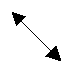D
Elastic (Ep>1)

Price EP=1P1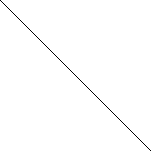On elastic (Ep<1 span="">EP=0

0 X1 C quantity demanded
Elasticity changing at different points of demand curve

From the above figure it can be seen that elasticity at point ‘C’ where the demand curve meets the quantity axis is equal to zero, and elasticity at point D where the demand curve meets the price axis is equal to infinity. If P1 is the mid-point of DC, elasticity at P1 is equal to 1. At all the points between P1 and C, the elasticity is greater than zero but less than unity and at the points between P1 and D elasticity is greater than unity but less than infinity. At point D, elasticity is equal to ∞. Thus the range of values of elasticity is between zero and infinity.

The elasticity computed at a single point on the demand curve for an infinitesimal change in price is called ‘point’ elasticity. The elasticity between two separate points of demand curve is called ‘arc’ elasticity. Point elasticity is more precise than arc elasticity concept.

The point elasticity is defined as the proportionate change in quantity demanded resulting from a very small change in price of that commodity. It is expressed as

Point Ep= - ∆Q/∆P x P/Q

Point elasticity can be calculated with the help of Differential Calculus.

Edp= dQ/dP x P/Q

Where Edp is point price elasticity of demand, dQ/dP is first order derivative of demand equation; P/Q is the ratio of price to quantity.

D1
Price of good A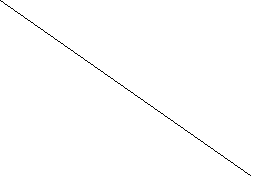P1 R1
P2 R2

0 Q1 Q2 D2 quantity of goods A
Point elasticity where the demand curve is linear

The above figure shows graphically where the point elasticity, in case of a linear demand curve, is the ratio of the segments of the line to the left of the particular point. The elasticity of the linear demand curve at point R1 is the ration of lower segment R1D2 to the upper segment R1D2 to the upper segment R1D1 that is,
R1D2 /R1D1
And at point R2, the elasticity is equal to R2D2/R2D1D1 Ep=∞

Ep>1 (elastic)

Ep=1

P1 Ep<1 inelastic="" span="">

D2 Ep=0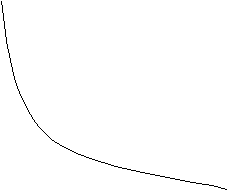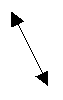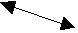0 Q1
Point elasticity where the demand curve is not linear

In the above figure the demand curve is not linear, determining point elasticity is simpler. From the figure it can be seen that any point to the right of point P1, elasticity is less than unity (Ep>1); where the demand curve touches the vertical axis Ep→∞And where the demand curve touches the horizontal axis Ep=0. thus the range of values of elasticity are 0≤Ep≤∞. To the right of P1, the demand is said to be inelastic (i.e. 0

Where Ep=0, the demand is perfectly inelastic
Ep=1, the demand is unity elasticity
Ep=∞ the demand is perfectly elastic

Arc elasticity:
Arc elasticity measures the average responsiveness to price change over a finite stretch on the demand curve. in the figure where MN refers to the stretch on the demand curve D1D2, it is not clear whether point M or point N should be considered to determine elasticity. It makes a difference from which point we start. Moving from point M to N is different from N to M. it is because the percentage changes in quantity and price is different, depending upon the price and quantity from which it is taken. The difference in the starting point reveals the different values of elasticity coefficients.

Price of good X D1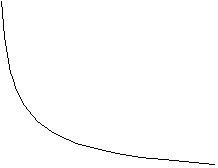6 M

N
3
D2

0 400 500 quantity demanded
Arc elasticity

The arc elasticity is defined as below:
ArcEp= ∆Q (P1+P2)/2 / ∆P (Q1+Q2)/2 = ∆Q (P1+P2)/∆P (Q1+Q2)
Where P1 and P2 are prices before and after changes Q1 and Q2 are quantities demanded before and after changes respectively. Is ∆Q and ∆P refer to change in the quantity demanded and change in the price respectively. To make the arc elasticity more meaningful, compute between the points on the demand curve that are close enough.
To conclude, elasticity of demand is a very important concept for decision makers whether it is a finance minister or a monopolist. The concept of elasticity of demand helps to measure the changes in the quantity demanded in relation to changes in income or price and analyze how price or income can be a decisive factor to bring desired change in the quantity demanded.

Tags:

## Search Entire Site

Custom Search